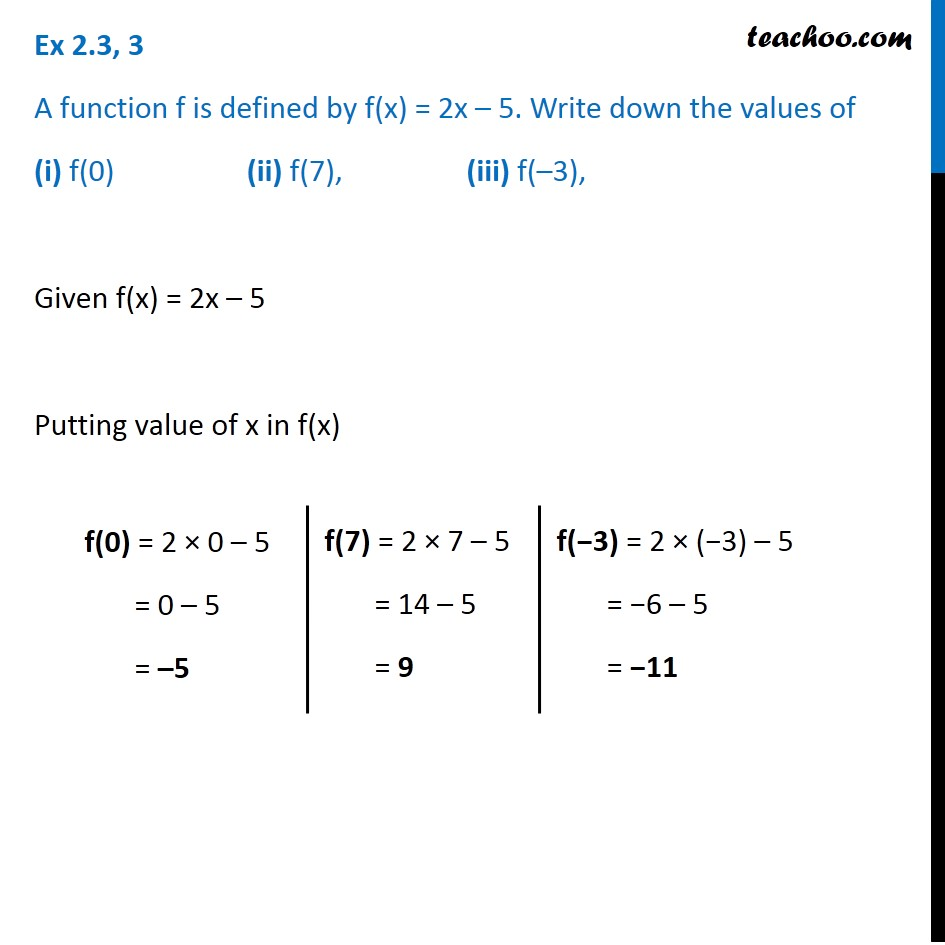Finding values at certain points

Chapter 2 Class 11 Relations and Functions
Concept wiseLearn in your speed, with individual attention - Teachoo Maths 1-on-1 Class

### Transcript

Ex 2.3, 3 A function f is defined by f(x) = 2x – 5. Write down the values of (i) f(0) (ii) f(7), (iii) f(–3), Given f(x) = 2x – 5 Putting value of x in f(x) f(0) = 2 × 0 – 5 = 0 – 5 = –5 f(7) = 2 × 7 – 5 = 14 – 5 = 9 f(−3) = 2 × (−3) – 5 = −6 – 5 = −11